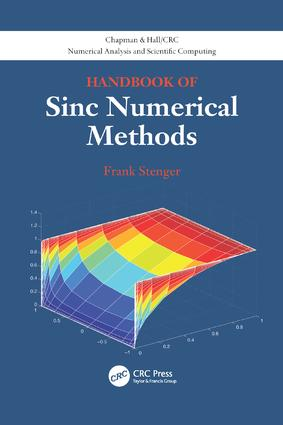# Handbook of Sinc Numerical Methods

## 1st Edition

CRC Press

482 pages | 56 B/W Illus.

##### Purchasing Options:\$ = USD
Paperback: 9781138116177
pub: 2017-05-31
\$82.95
x
Hardback: 9781439821589
pub: 2010-12-02
\$220.00
x
eBook (VitalSource) : 9780429104046
pub: 2016-04-19
from \$39.98

FREE Standard Shipping!

### Description

Handbook of Sinc Numerical Methods presents an ideal road map for handling general numeric problems. Reflecting the author’s advances with Sinc since 1995, the text most notably provides a detailed exposition of the Sinc separation of variables method for numerically solving the full range of partial differential equations (PDEs) of interest to scientists and engineers. This new theory, which combines Sinc convolution with the boundary integral equation (IE) approach, makes for exponentially faster convergence to solutions of differential equations. The basis for the approach is the Sinc method of approximating almost every type of operation stemming from calculus via easily computed matrices of very low dimension.

The CD-ROM of this handbook contains roughly 450 MATLAB® programs corresponding to exponentially convergent numerical algorithms for solving nearly every computational problem of science and engineering. While the book makes Sinc methods accessible to users wanting to bypass the complete theory, it also offers sufficient theoretical details for readers who do want a full working understanding of this exciting area of numerical analysis.

### Reviews

The author, a well-known expert in this area, has published many papers dealing with various aspects of sinc methods. A key result is that sinc methods can converge very fast under certain assumptions on the given problem. … practical aspects are covered in great detail. In particular, there is an accompanying CD-ROM that contains about 450 MATLAB programs where sinc methods are implemented to solve various problems. The book provides a good description of these programs, so a user with a certain equation to solve can easily find an appropriate sinc algorithm. … it should be useful reading for practitioners who have heard about sinc methods and want to use them.

—Kai Diethelm, Computing Reviews, September 2011

One-Dimensional Sinc Theory

Introduction and Summary

Sampling over the Real Line

More General Sinc Approximation on R

Sinc, Wavelets, Trigonometric and Algebraic Polynomials and Quadratures

Sinc Methods on Γ

Rational Approximation at Sinc Points

Polynomial Methods at Sinc Points

Sinc Convolution-BIE Methods for PDE and IE

Introduction and Summary

Some Properties of Green’s Functions

Free-Space Green’s Functions for PDE

Laplace Transforms of Green’s Functions

Multi-Dimensional Convolution Based on Sinc

Theory of Separation of Variables

Explicit 1-d Program Solutions via Sinc-Pack

Introduction and Summary

Sinc Interpolation

Approximation of Derivatives

Sinc Indefinite Integration

Sinc Indefinite Convolution

Laplace Transform Inversion

Hilbert and Cauchy Transforms

Sinc Solution of ODE

Wavelet Examples

Explicit Program Solutions of PDE via Sinc-Pack

Introduction and Summary

Elliptic PDE

Hyperbolic PDE

Parabolic PDE

Performance Comparisons

Directory of Programs

Wavelet Formulas

One Dimensional Sinc Programs

Multi-Dimensional Laplace Transform Programs

Bibliography

Index

### About the Author

Frank Stenger is a professor emeritus at theUniversity of Utah, where he received the distinguished research award. One of the leading contributors to the area of numerical analysis, Dr. Stenger is the main developer of Sinc numerical methods and has authored over 160 papers in various journals.

### Subject Categories

##### BISAC Subject Codes/Headings:
MAT003000
MATHEMATICS / Applied
MAT007000
MATHEMATICS / Differential Equations
MAT021000
MATHEMATICS / Number Systems